# Simulating Stock Prices Using Geometric Brownian Motion

Disclaimer: This project/post is for fun/education please don’t use the results of this project to make investment decisions. If you chose to ignore this disclaimer and do just that I am not responsible for the (very probable) large losses that may occur.

A stochastic process, S, is said to follow Geometric Brownian Motion (GBM) if it satisfies the stochastic differential equation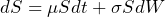where

•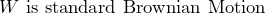•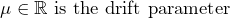•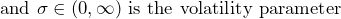For an arbitrary starting value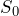, the SDE has the analytical solution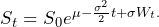Standard BM models multiple phenomena. For example, the paths assumed by gas particles as they interact with nearby particles follow a Brownian motion. However, for some applications standard BM is insufficient due to the potential of the process to be negative. Because GBM is the exponential of standard BM with drift parameter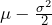and scale parameter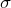the process is a BM that cannot be negative. This makes it ideal for applications in economics and finance.

In a previous post, I naively modeled stock price movements with movements drawn from normal distributions parameterized by the statistical data of past movements in the stock price. Monte Carlo methods were employed to generate multiple paths and best-, worst-, and average-case results were determined from the simulated paths. In this post, I will be doing much of the same except the stock price movements will be more accurately simulated with GBM. As mentioned above, paths following a GBM are more standard in economics and finance.

I will also share a tool that uses these simulations to determine the probability of a stock price reaching certain price points at a particular time in the future. One main application for such an analysis of the simulated paths is to help in determining the probability of profit when buying and selling stock options. Tools like these are available from most brokerage firms (e.g. Ally Invest and Merril Edge) and are likely much more complicated than the one I’m offering (if the one offered by your brokerage firm isn’t, you should consider finding a new brokerage firm) however, I thought it would be interesting to develop my own to better understand the tools and GBM.

## Stock Price Paths With GBM

Much of the code presented here is stolen from my previous project described above. The only real difference is in the generation of the paths where GBM is substituted for the paths drawn from a few normal distributions with different parameterizations. Most of the Monte Carlo simulations, plotting, and result display is shared between the two projects.

To create simulations of future stock prices using GBM we will first need to gather historical stock price data for the ticker symbols we’re interested in. To get this data the Yahoo Finance API that I developed in a previous post will be used. Installation and usage information for this API is available on its GitHub page.

The following must be done to generate a potential stock price path:

1. retrieve historical data
2. determine the drift and volatility parameters for the BM
3. determine random shocks for each time step in the forecast horizon
4. build the BM which incorporates all previous shocks to the initial stock price
5. and finally, plug the BM values into the GBM equation above to generate a stock path

This can be seen more easily in the Python code. Before we get that far though we need to develop a class to hold information about the stock and its price data. In this project, this class is very simple and is more of a remnant from the previous naive attempt. My goal here was to use most of the same code and that included the Stock class.

# stock.py - Stock class to hold data about the stock
#                    and its price data
import pandas as pd

class Stock(object):
def __init__(self, symbol, data):
self.symbol = symbol
self.data = data
self.data.Date = pd.to_datetime(self.data.Date, format="%Y-%m-%d")
self.compute_stats()

# daily precentage change

# average daily change (%)
self.avg = self.open_diffs.mean()
# variance of daily percentage changes
self.var = self.open_diffs.var()
# standard deviation of daily percentage changes
self.std = self.open_diffs.std()

def get_sym(self):
return self.symbol

def get_daily_return_avg(self):
return self.avg

def get_daily_return_variance(self):
return self.var

def get_daily_return_std(self):
return self.std


The class above is very straightforward and helps to minimize the lines of code in the base simulation file. The part of the simulation logic used for generating stock paths is provided below.

def run(self, ticker_list, simulations, final_day, data_months=1, plot_results=True):
# number of paths
self.simulations = simulations
# last day of simulation
self.final_day = datetime.datetime.strptime(final_day, "%Y-%m-%d")
# number of months worth of data to use
self.data_months = data_months
self.current = datetime.datetime.today()

self.T = (self.final_day - self.current).days + 2
if self.T <= 0:
print("Final day is on or after the current day.")
exit()

# weekends don't exist
self.removed_dates = []
for d in range(self.T):
date = (self.current + relativedelta(days=d))
if date.weekday() > 4:
self.removed_dates.append(date)
self.T -= 1

dh = YahooFinanceAPI(interval=Interval.DAILY)
symbols = ticker_list
portfolio = {}

# get data for all of the tickers
past = self.current - relativedelta(days=int(self.data_months*30))
portfolio = dh.get_data_for_tickers(symbols, past, self.current)
for sym in symbols:
# create Stock objects for each ticker
portfolio[sym] = Stock(sym, portfolio[sym])

paths = {}
for sym in symbols:
stock = portfolio[sym]
s_0 = stock.get_last_price()
sig = stock.get_daily_return_std()
sig2 = sig**2
mu = stock.get_daily_return_avg()

# random daily shocks to the stock price (price fluctuations)
shocks = {sim: random.normal(0, 1, self.T) for sim in range(self.simulations)}
# cumulative sum of all previous shocks to the stock price (price path)
brownian_motion = {sim: shocks[sim].cumsum() for sim in range(self.simulations)}
paths[stock.get_sym()] = [[s_0] for _ in range(self.simulations)]

# create a simulated price path using the stock price's
# statistics and the random shocks from above
for i in range(self.simulations):
paths[stock.get_sym()][i].extend(\
[s_0 * np.exp(((mu - 0.5*sig2)*j) + sig*brownian_motion[i][j-1]) for j in range(1, self.T+1)]
)

# defined below in Full Code section
if plot_results:
self.plot(paths, portfolio, symbols)
return paths

The code snippet above is the run(…) function from the GBMMonteCarlo class. This code uses movements determined by GBM to produce multiple possible trajectories a stock price can take (more on the multiple paths below). First, the historical data is retrieved for each ticker symbol via the YahooFinanaceAPI package. The statistics, particularly the mean and variance, of the daily percentage movements of the stock price data is computed automatically when creating the Stock objects from the data. These statistics are used to parameterize the GBM with the mean being the drift parameter and the standard deviation being the volatility. With these parameters the final stock price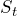is determined by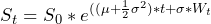where

•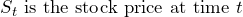•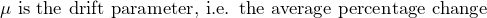•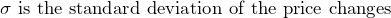•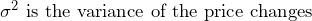•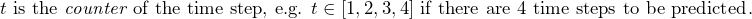•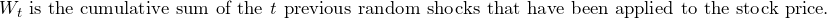In my opinion, these variables are most easily understood by reading and understanding the code snippet. Printing the values for different parameterizations of the simulation can help build an intuition of how the process is working. Since we’re dealing with a dt value of 1 (one day per time step), there is no t value as seen in the equation. Rather, the number of days between the start and end date is calculated and a looping variable j is used as the t parameter in the logic.

### Monte Carlo Method

The description below is shamelessly stolen from a previous (very similar) post of mine.

Monte Carlo simulation is a way to model a stochastic process that cannot easily be predicted due to underlying sources of randomness. Monte Carlo simulations are widely used in many fields including energy, engineering, insurance, oil and gas transportation, and, as in our case, finance. These simulations provide risk analysis by building many different models of the process with different random values drawn from a (or a set of) probability distributions. In the case of stock price predictions, we are essentially creating many different paths the stock can take as time progresses and determining what an average path might look like.

As seen in the code snippet above, more than one path is generated when running this logic. This is the essence of Monte Carlo simulations. One parameter of this project is the number of simulations to use for determining the future price of a stock. This simulations parameter determines the number of paths to be generated by our stochastic process.

In this case, one thing we can learn from the Monte Carlo simulations is the likelihood of the stock falling below or rising above specified stock prices. Determining these likelihoods forms the basis of the options buying tool presented below.

### Results

By way of example, the Monte Carlo simulations will be run for two stock tickers, MLR (Miller Industries) and GNTX (Gentex), with a 1-month forecast horizon. There will be 50 paths generated and 2 month’s worth of historical data is used to determine the drift and volatility parameters. Programmatically, running the simulation (provided the GBMMonteCarlo() class) would look something like this

if __name__ == '__main__':
gbm_mc = GBMMonteCarlo()
gbm_mc.run(["mlr", "gntx"], 50, '2020-10-29', data_months=2)

Recalling that the positional parameters to the run() method are ticker_list, simulations, and final_day. Running this simulation with plotting enabled (i.e. the plot_results parameters is set to True) produces the following graphic.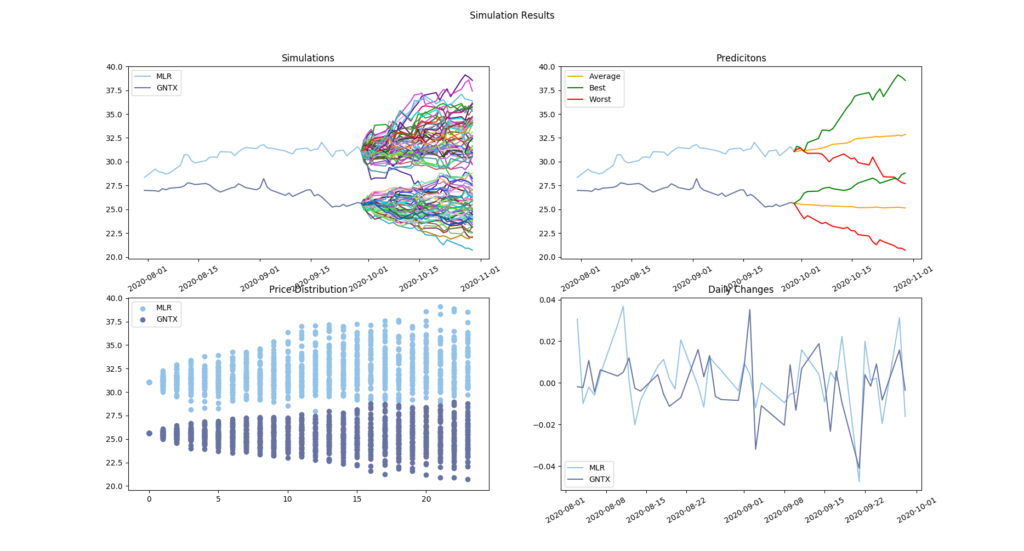50 simulated paths for ticker symbols GNTX and MLR. The predicted days range from 2020-09-29 to 2020-10-29.

Note that we’re using a small number of simulations here so the results can more easily be seen in the graphic. I’m also going to point out how bad the axis labels look, it’s a little difficult to get it all to fit correctly when the x-axis labels are dates.

Seen here are the 50 individual paths following GBM. When looking at the first two graphs, the past data for GNTX appears to be on a downward trend and, in fact, this downward trend is seen to continue in the average (yellow) case in the top-right graph. The opposite can be seen in the same graphs but for the ticker symbol MLR which is trending upward. The bottom two graphs give an idea of how the prices of the simulations are distributed each predicted day. The lower left helps show the range of prices each day and how they cluster. The bottom right shows the historical daily percent changes in the stock price for the 2 months of historical data used in this simulation.

Stock options are contracts that give the buyer the right, but not the obligation, to buy or sell an underlying asset at a specified price on or before a specified date (for American options, which are most typical). The specified price is known as the strike price and the specified date is known as the expiration date. There are two types of options: call options and put options. The owner of a call option has the right, but not the obligation, to buy 100 shares of the underlying asset at the strike price of the option on or before the option expires. Conversely, a put option gives the owner the right, but not the obligation to sell 100 shares of the underlying asset at the strike price on or before the option expires.

There are many different option trading strategies. Two basic (and risky) strategies are to buy call options if you think the price of the option and the underlying asset is going up (they typically move together) or to buy put options if you think the underlying asset will decrease in value (which usually causes a put option to become more valuable). The purpose of this post isn’t to discuss option trading strategies. I’ve given this information here to provide some insight into the utility of having some (probabilistic) idea of where a stock will be trading at some time in the future.

This leads us to the most common question asked when trading options: what is the likelihood of a stock rising above or falling below a particular price (typically the strike price)? For some strategies, the trader would like to know the likelihood of the stock falling between two different strikes prices. A 100% accurate answer to these questions is the golden ticket since it would eliminate all of the risks of investing in these assets (or in the stocks themselves). Unfortunately, due to the nature of the stock market and the speed at which (sometimes randomly distributed) information propagates this is an impossible task. However, we can use the Monte Carlo simulation engine developed above to get at least somewhat of an answer to this question.

To do this we will need some information about the assets underlying the options. Particularly, we will need the ticker symbols (to get the historical data), the high strike price for each ticker (the price we want the stock to stay [or fall] under), and the low strike price for each ticker (the price we want to stock to stay [or rise] above). With this info, we will run the GBM-MC simulation and then compute the statistics of what the stock price is on expiration. This is done in the logic below.

if __name__ == '__main__':
sims = 500
mc_sim = GBMMonteCarlo()
ticker_list = ["ge", "intc", "gntx", "INO"]
# get results for each ticker
results = mc_sim.run(ticker_list, sims, '2020-10-23', data_months=0.5, plot_results=False)

# high and low strikes for each ticker
high_strike_price_dict = {"ge": 6.5, "intc": 52.0, "gntx": 26.0, "INO": 10.50}
low_strike_price_dict = {"ge": 6.0, "intc": 48.0, "gntx": 22.0, "INO": 10.0}
for sym in ticker_list:
# compute the likelihood of ending above, below, or under
# high and low strikes.
above_high, below_high = option_probability(sims, results[sym], high_strike_price_dict[sym])
above_low, below_low = option_probability(sims, results[sym], low_strike_price_dict[sym])
between = above_low - above_high

# display the results
prt_str = "{} Low Price: {}  High Price: {} \
\n\tBelow Low: {}  Above Low: {}  Between: {}  Below High: {}  Above High: {} \
\n\tBelow Low: {:.2f}%  Above Low: {:.2f}%  Between: {:.2f}%  Below High: {:.2f}%  Above High: {:.2f}%\n\n"
print(prt_str.format(sym.upper(), low_strike_price_dict[sym], high_strike_price_dict[sym],
below_low, above_low, between, below_high, above_high, (below_low/sims)*100,
(above_low/sims)*100, (between/sims)*100, (below_high/sims)*100, (above_high/sims)*100))

To compute the statistics (number of prices above/below the high/low strikes) the following option_probability() function is used.

def option_probability(simulations, results, strike_price):
"""
Get the number of simulations above and below
a particular strike price.
"""
below_count = 0
above_count = 0
last_idx = len(results) - 1
for i in range(simulations):
price = results[i][last_idx]
if price < strike_price:
below_count += 1
else:
above_count += 1

return above_count, below_count

Running the code above produces the following output for these 4 ticker symbols:

GE Low Price: 6.0  High Price: 6.5
Below Low: 23  Above Low: 27  Between: 11  Below High: 34  Above High: 16
Below Low: 46.00%  Above Low: 54.00%  Between: 22.00%  Below High: 68.00%
Above High: 32.00%

INTC Low Price: 48.0  High Price: 52.0
Below Low: 4  Above Low: 46  Between: 13  Below High: 17  Above High: 33
Below Low: 8.00%  Above Low: 92.00%  Between: 26.00%  Below High: 34.00%
Above High: 66.00%

GNTX Low Price: 22.0  High Price: 26.0
Below Low: 16  Above Low: 34  Between: 33  Below High: 49  Above High: 1
Below Low: 32.00%  Above Low: 68.00%  Between: 66.00%  Below High: 98.00%
Above High: 2.00%

INO Low Price: 10.0  High Price: 10.5
Below Low: 31  Above Low: 19  Between: 3  Below High: 34  Above High: 16
Below Low: 62.00%  Above Low: 38.00%  Between: 6.00%  Below High: 68.00%
Above High: 32.00%

The output above gives, based on the simulated stock price paths, the likelihood that the stock price will fall above, below, or between the high in low strike prices. Theoretically, more simulations and more accurate estimates of volatility and drift for the GBM will give more realistic results. Of course, this is all assuming the estimates for the volatility and drift parameters remain constant from the current time to the expiration date. This is unlikely to be the case if the underlying company release information that investors find particularly good or particularly bad (which is why, in my opinion, an investor should try to stick to limited loss options strategies, although these typically limit profits as well).

### Project Website

This tool can be found on my projects webpage under Finance > Options Buying Tool making it easier to use without having to implement the project yourself. Below is an example of the interface which shows how to run the simulation as well as a screenshot of the results you can expect. The same tickers, strikes, simulation count, and dates as above are reused for consistency.

The screenshot above shows how the tool expects you to enter the parameters. On the project’s webpage, there are some instructions and descriptions for these fields but, essentially, you enter a ticker symbol, high strike price, and low strike price for each stock you want to evaluate. The remainder of the parameters are for the simulation and are discussed to some extent above and on the webpage. Clicking the simulate button will run the simulations and produce output like the that shown below (I’m sorry if you experience long wait times, I’m working on optimization continuously).

This is essentially the same output as the script but in a more appealing format (arguably).

## Conclusion

Presented here is a look at GBM and how it can be used to simulate stock prices. As an added benefit, these simulated prices have been applied to the real-world application of determining the probability of a stock ending above, below, or between two different prices at some expiration date. As mentioned in the introduction tools like these should be available from your brokerage firm and should work much better than the one presented here. Given the choice, you should obviously stick to the professional tools. However, this post illuminates how these mathematics coupled with some Python programming can provide a pretty useful tool for an options trader.

## Full Code

#### stock.py

import pandas as pd

class Stock(object):
def __init__(self, symbol, data):
self.symbol = symbol
self.data = data
self.data.Date = pd.to_datetime(self.data.Date, format="%Y-%m-%d")
self.compute_stats()

self.avg = self.open_diffs.mean()
self.var = self.open_diffs.var()
self.std = self.open_diffs.std()

def get_sym(self):
return self.symbol

#### simulation.py

from yfapi import YahooFinanceAPI, Interval
from stock import Stock

import datetime
import matplotlib.pyplot as plt
import pandas as pd
from numpy import random
import numpy as np
from dateutil.relativedelta import relativedelta
from numpy.fft import fft

class GBMMonteCarlo(object):
def __init__(self):
pass

def run(self, ticker_list, simulations, final_day, data_months=1, plot_results=True):
self.simulations = simulations
self.final_day = datetime.datetime.strptime(final_day, "%Y-%m-%d")
self.data_months = data_months
self.current = datetime.datetime.today()
self.T = (self.final_day - self.current).days + 2

if self.T <= 0:
print("Final day is on or after the current day.")
exit()

self.removed_dates = []
for d in range(self.T):
date = (self.current + relativedelta(days=d))
if date.weekday() > 4: #or (date in holidays):
self.removed_dates.append(date)
self.T -= 1

dh = YahooFinanceAPI(interval=Interval.DAILY)
symbols = ticker_list
portfolio = {}

past = self.current - relativedelta(days=int(self.data_months*30))
portfolio = dh.get_data_for_tickers(symbols, past, self.current)
for sym in symbols:
portfolio[sym] = Stock(sym, portfolio[sym])

paths = {}
for sym in symbols:
stock = portfolio[sym]
s_0 = stock.get_last_price()
sig = stock.get_daily_return_std()
sig2 = sig**2
mu = stock.get_daily_return_avg()

# random daily shocks to the stock price (price fluctuations)
shocks = {sim: random.normal(0, 1, self.T) for sim in range(self.simulations)}
# cumulative sum of all previous shocks to the stock price (price path)
brownian_motion = {sim: shocks[sim].cumsum() for sim in range(self.simulations)}
paths[stock.get_sym()] = [[s_0] for _ in range(self.simulations)]

for i in range(self.simulations):
paths[stock.get_sym()][i].extend(\
[s_0 * np.exp(((mu - 0.5*sig2)*j) + sig*brownian_motion[i][j-1]) for j in range(1, self.T+1)]
)

if plot_results:
self.plot(paths, portfolio, symbols)
return paths

def plot(self, paths, portfolio, symbols):
max_idx_dict = {}
min_idx_dict = {}

for sym in symbols:
max_amt = -1000000000
min_amt = 1000000000
last_idx = len(paths[sym]) - 1

for i in range(self.simulations):
price = paths[sym][i][last_idx]
if price < min_amt: # lowest final price
min_amt = price
min_idx_dict[sym] = i
if price > max_amt: # highest final price
max_amt = price
max_idx_dict[sym] = i

avg_price_dict = {}
for sym in symbols:
avg_price_dict[sym] = [portfolio[sym].data.Close[len(portfolio[sym].data) - 1]]
for i in range(self.T):
avg = 0.0
for j in range(self.simulations):
avg += paths[sym][j][i]
avg_price_dict[sym].append(float(avg)/float(self.simulations))

# plot the simulation data
fig, (ax1, ax2) = plt.subplots(2, 2)

dates = portfolio[sym].data.Date.tolist()
new_dates = [dates[-1]]
new_dates.extend(pd.date_range(start=self.current, end=(self.final_day + relativedelta(days=1))).tolist())
new_dates = [date for date in new_dates if date not in self.removed_dates]

fig.suptitle("Simulation Results")
colors = [np.random.rand(3,) for _ in range(len(symbols))]
count = 0
for sym in symbols:
open = portfolio[sym].data.Close.tolist()

ax1.plot(dates, open, color=colors[count], label=sym.upper())
for i in range(self.simulations):
ax1.plot(new_dates, paths[sym][i], color=np.random.rand(3, ))

ax1.plot(dates, open, color=colors[count])
ax1.plot(new_dates, avg_price_dict[sym], color="orange", label="Average")
ax1.plot(new_dates, paths[sym][max_idx_dict[sym]], color="green", label="Best")
ax1.plot(new_dates, paths[sym][min_idx_dict[sym]], color="red", label="Worst")

x = [range(self.T+1) for _ in range(self.simulations)]
ax2.scatter(x, paths[sym], color=colors[count], label=sym.upper())

ax2.plot(portfolio[sym].data.Date[1:], portfolio[sym].open_diffs, \
color=colors[count], label=sym.upper())

if count == 0:
ax1.legend()
ax1.set_title("Predicitons")

if count == (len(symbols) -1):
ax1.legend()
ax1.set_title("Simulations")
ax2.legend()
ax2.set_title("Price Distribution")
ax2.set_title("Daily Changes")
ax2.legend()

ax1.tick_params(axis='x', labelrotation=30)
ax1.tick_params(axis='x', labelrotation=30)
ax2.tick_params(axis='x', labelrotation=30)

count += 1
plt.show()

if __name__ == '__main__':
gbmmc = GBMMonteCarlo()
gbmmc.run(["mlr", "gntx"], 50, '2020-10-29', data_months=2)

#### options_tool.py

#!/usr/bin/python3
from simulation import GBMMonteCarlo

def option_probability(simulations, results, strike_price):
below_count = 0
above_count = 0
last_idx = len(results) - 1
for i in range(simulations):
price = results[i][last_idx]
if price < strike_price:
below_count += 1
else:
above_count += 1

return above_count, below_count

if __name__ == '__main__':
sims = 50

mc_sim = GBMMonteCarlo()
ticker_list = ["ge", "intc", "gntx", "INO"]
results = mc_sim.run(ticker_list, sims, '2020-10-23', data_months=0.5, plot_results=False)

high_strike_price_dict = {"ge": 6.5, "intc": 52.0, "gntx": 26.0, "INO": 10.50}
low_strike_price_dict = {"ge": 6.0, "intc": 48.0, "gntx": 22.0, "INO": 10.0}
for sym in ticker_list:
above_high, below_high = option_probability(sims, results[sym], high_strike_price_dict[sym])
above_low, below_low = option_probability(sims, results[sym], low_strike_price_dict[sym])
between = above_low - above_high

prt_str = "{} Low Price: {}  High Price: {} \
\n\tBelow Low: {}  Above Low: {}  Between: {}  Below High: {}  Above High: {} \
\n\tBelow Low: {:.2f}%  Above Low: {:.2f}%  Between: {:.2f}%  Below High: {:.2f}%  Above High: {:.2f}%\n\n"
print(prt_str.format(sym.upper(), low_strike_price_dict[sym], high_strike_price_dict[sym],
below_low, above_low, between, below_high, above_high, (below_low/sims)*100,
(above_low/sims)*100, (between/sims)*100, (below_high/sims)*100, (above_high/sims)*100))